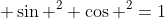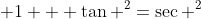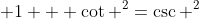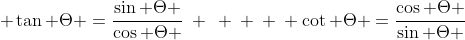# Trig Identities Cheat Sheet

## Trig Identities Cheat Sheet

This is your trig identities cheat sheet!  Trig identities are defined as mathematical expressions that relate various trigonometric functions to others, regardless of what the variables are.  The trig identities are true for all values of the variable.  They are incredibly valuable to understand and to memorize, and their usefulness becomes apparent when you are faced with a complicated trigonometric expression that needs to be simplified.

All you need to do is rearrange the complicated trig expression such that you can express it in terms of the trigonometric identities, substitute in the identity to simplify, and carry on with solving the math problem.  Think of them as a type of "constant" that can be swapped into expressions (that use sine, cosine, tangent, or any of the related trig functions secant, cosecant, or cotangent) to change their appearance without changing the math surrounding the expression.

Trig identities are extraordinarily important in helping you solve your mathematics questions, and so the identities that I list and explain on this trig identities cheat sheet should really be committed to memory.  If you know them and can recognize them automatically, your math homework and trigonometry questions will become a lot easier.

### Reciprocal Identities

For the first set of trig identities, I will list the Reciprocal Identities.  These may be familiar to you already, especially if you have been introduced to the trig functions secant, cosecant, and cotangent.  I'll refer you to my post on sinecosine, and tangent for their basic definitions, as I will build upon those.  If you look at the definition of sine, you see that it is the ratio of opposite over hypotenuse.  (Remember SOHCAHTOA!)  If you invert this ratio to be hypotenuse over opposite, this is the definition of cosecant.  The same thing applies to secant and cotangent: they are the reciprocal ratio of the sides that define the basic trig identity.  So, then you can also say that these trig functions are the reciprocal of the basic trigonometric functions.  Here they are listed for you:

Naturally, you can rearrange these trig identities to isolate the other trigonometry functions as well:

And those are the first set of trig identities I am going to mention here on my trig identities cheat sheet: the Reciprocal Identities.

### Pythagorean Identities

The next set that I would like to mention are the Pythagorean Identities.  Allow me to direct you to a recent post I put up on my In Mathematics blog that had a lengthy explanation about Pythagorean Identities.  I recommend giving that a read to better understand where these trig identities are coming from and how they can be derived.  However, for completeness, I will list them here as well, like above.### Quotient Identities

The Quotient Identities are another set of trig identities that are fairly easy to remember, especially when you understand how to derive them.  Start with the basic trig definitions for sine and cosine.  Sine is opposite over hypotenuse, cosine is adjacent over hypotenuse.  Now, divide sine by cosine:

sine / cosine = [opposite / hypotenuse] / [adjacent / hypotenuse] = opposite / hypotenuse

The hypotenuse in the numerator and denominator expressions cancel out, and you are left simply with the basic trigonometric definition of tangent!  Similarly,divide cosine by sine to get the second quotient identity.The identities that I have explained above are sometimes considered to be the 8 fundamental trigonometric identities, including the Reciprocal (x3), Pythagorean (x3), and Quotient (x2) identities. Hopefully my explanations have helped you to understand the logic behind these identities, which will ideally make them easier for you to memorize and work with!

Please remember to +1 me if this was useful!

#### 1 comment:

1.This comment has been removed by a blog administrator.